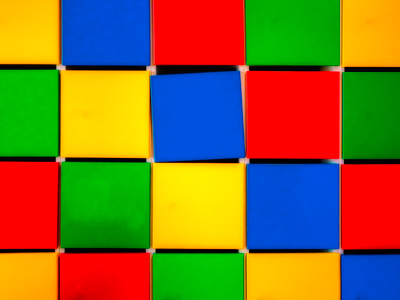If you double the length of a square’s sides, its area increases fourfold.

# Perimeter and Area 3 (Medium)

Welcome to the third in our Medium Difficulty series of Eleven Plus maths quizzes on perimeter and area. If you have played our previous quizzes, then you should be familiar with some of the formulae we use to work out the perimeter and area of 2D shapes. Here we’ll give you more practice, and throw in the odd 3D shape as well.

When dealing with circles, you will need to use π (pi). This is a mathematical constant used to calculate the circumference and the area of circles. Do you know its value? Some people can recite it to hundreds of decimal places, but three is generally enough!

Here’s pi to 100 decimal places, just in case you'd like to memorise it:

3.141592653589793238462643383279502884197169399375105820974
9445923078164062862089986280348253421170679

Because pi is an unusual number, you may want to use your calculator in this quiz. If so, don’t use the button marked π. Any questions you need pi for will tell you the value to use.

Now for the quiz – good luck!

1.
Jane is tiling her bathroom wall. The area of the wall is 8 m2. Each tile has an area of 400 cm2. How many tiles will Jane need?
20
200
400
4,000
First, we need to find out how many square cm are in a square metre: 100 x 100 = 10,000
Next we divide 10,000 by 400 to find how many tiles are needed to cover one square metre: 10,000 ÷ 400 = 25
If it takes 25 tiles to cover 1 m2, then it will take 25 x 8 to cover 8 m2: 25 x 8 = 200
Jane will need 200 tiles – as long as she doesn’t break any that is!
2.
The area of a right-angled triangle is 108 cm2. If its base is 12 cm, what is its height?
9 cm
13 cm
15 cm
18 cm
Area = (base × height) ÷ 2:
108 = (12 x height) ÷ 2:
2 x 108 ÷ 12 = height:
216 ÷ 12 = height = 18 cm
3.
If the diameter of a circle is 24 cm, what is its circumference (assume that π = 3.142 and round your answer to the nearest whole number)?
45 cm
55 cm
65 cm
75 cm
The circumference of a circle = 2πr. As you know, diameter = 2r so part of this problem was already done for you!
4.
Circle A has an area of 12 cm2. Circle B has a diameter twice that of circle A. What is the area of circle B?
24 cm2
36 cm2
48 cm2
64 cm2
If the diameter of a circle doubles, its area quadruples.
Diameter is twice radius. In circles, area = πr2. If r = 1 cm, A = 3.142 × 1 × 1 = 3.142 cm. Now, if r = 2, A = 3.142 × 2 × 2 = 12.568.
It’s not obvious at first but 12.568 is four times 3.142. Work it out and see for yourself.
5.
The surface area of a cube is 54 cm2. How tall is the cube?
3 cm
4 cm
5 cm
6 cm
A cube has six faces: each face is a square. So, to find the area of each face, divide 54 by 6:
54 ÷ 6 = 9. Next, we want to find the length of each square. The formula for the area of a square is: Area = length2
If you know your times tables then you should know that 3 x 3 = 9
6.
If the circumference of a circle is 19 cm, what is its radius (assume that π = 3.142 and round your answer to the nearest whole number)?
2 cm
3 cm
4 cm
5 cm
Circumference = 2πr, so 19 = 2 × 3.142 × r
We can rearrange the problem to, r = 19 ÷ (3.142 x 2):
So, r = 19 ÷ 6.284 = 3.024 which we round down to 3 cm
7.
Square A has an area of 13 cm2. Square B has sides twice as long as those of square A. What is the area of square B?
26 cm2
52 cm2
39 cm2
65 cm2
You didn’t need to work out the side lengths of square A to find this answer – if you double the length of a square’s sides, its area increases fourfold. This should help you to understand:
Suppose we have a square of side length 2 cm. Its area = 2 x 2 = 4 cm2
Now we double the length of its sides. Its area is now 4 x 4 = 16 cm2
16 ÷ 4 = 4. The area has quadrupled in size
8.
The area of a square is 64 cm2. What is its perimeter?
8 cm
16 cm
32 cm
48 cm
The area of a square is length x length. 8 x 8 = 64
The perimeter of a square is 4 x its length. 8 x 4 = 32
9.
A regular octagon has a perimeter of 108 cm. How long are each of its sides?
16.5 cm
15.5 cm
14.5 cm
13.5 cm
An octagon has eight sides (like an octopus has eight legs). A regular octagon has ALL its sides the same length ∴ perimeter = 108 ÷ 8 = 13.5 cm
10.
What is the area of a circle whose radius is 10 cm (assume π = 3.142)?
314.2 cm2
31.42 cm2
3,142 cm2
3.142 cm2
The formula for finding the area of a circle is Area = π × r2
π x 102 = 3.142 x 100 = 314.2 cm2
Author:  Frank Evans